JMSLTM Numerical Library 7.2.0
com.imsl.stat

## Class MultiCrossCorrelation

• All Implemented Interfaces:
Serializable, Cloneable

```public class MultiCrossCorrelation
extends Object
implements Serializable, Cloneable```
Computes the multichannel cross-correlation function of two mutually stationary multichannel time series.

`MultiCrossCorrelation` estimates the multichannel cross-correlation function of two mutually stationary multichannel time series. Define the multichannel time series X by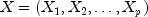where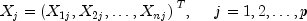with n = `x.length` and p = `x.length`. Similarly, define the multichannel time series Y by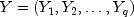where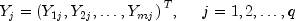with m = `y.length` and q = `y.length`. The columns of X and Y correspond to individual channels of multichannel time series and may be examined from a univariate perspective. The rows of X and Y correspond to observations of p-variate and q-variate time series, respectively, and may be examined from a multivariate perspective. Note that an alternative characterization of a multivariate time series X considers the columns to be observations of the multivariate time series while the rows contain univariate time series. For example, see Priestley (1981, page 692) and Fuller (1976, page 14).

Let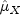= `xmean` be the row vector containing the means of the channels of X. In particular,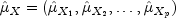where for j = 1, 2, ..., p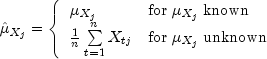Let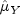= `ymean` be similarly defined. The cross-covariance of lag k between channel i of X and channel j of Y is estimated by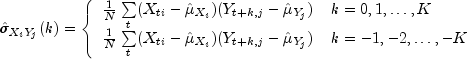where i = 1, ..., p, j = 1, ..., q, and K = `maximum_lag`. The summation on t extends over all possible cross-products with N equal to the number of cross-products in the sum.

Let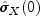= `xvar`, where `xvar` is the variance of X, be the row vector consisting of estimated variances of the channels of X. In particular,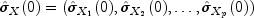where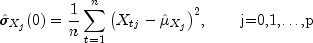Let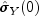= `yvar`, where `yvar` is the variance of Y, be similarly defined. The cross-correlation of lag k between channel i of X and channel j of Y is estimated by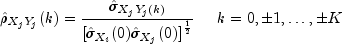Example, Serialized Form
• ### Nested Class Summary

Nested Classes
Modifier and Type Class and Description
`static class ` `MultiCrossCorrelation.NonPosVariancesException`
The problem is ill-conditioned.
• ### Constructor Summary

Constructors
Constructor and Description
```MultiCrossCorrelation(double[][] x, double[][] y, int maximum_lag)```
Constructor to compute the multichannel cross-correlation function of two mutually stationary multichannel time series.
• ### Method Summary

Methods
Modifier and Type Method and Description
`double[][][]` `getCrossCorrelation()`
Returns the cross-correlations between the channels of `x` and `y`.
`double[][][]` `getCrossCovariance()`
Returns the cross-covariances between the channels of `x` and `y`.
`double[]` `getMeanX()`
Returns the mean of each channel of `x`.
`double[]` `getMeanY()`
Returns the mean of each channel of `y`.
`double[]` `getVarianceX()`
Returns the variances of the channels of `x`.
`double[]` `getVarianceY()`
Returns the variances of the channels of `y`.
`void` `setMeanX(double[] mean)`
Estimate of the mean of each channel of `x`.
`void` `setMeanY(double[] mean)`
Estimate of the mean of each channel of `y`.
• ### Methods inherited from class java.lang.Object

`clone, equals, finalize, getClass, hashCode, notify, notifyAll, toString, wait, wait, wait`
• ### Constructor Detail

• #### MultiCrossCorrelation

```public MultiCrossCorrelation(double[][] x,
double[][] y,
int maximum_lag)```
Constructor to compute the multichannel cross-correlation function of two mutually stationary multichannel time series.
Parameters:
`x` - A two-dimensional `double` array containing the first multichannel stationary time series. Each row of `x` corresponds to an observation of a multivariate time series and each column of `x` corresponds to a univariate time series.
`y` - A two-dimensional `double` array containing the second multichannel stationary time series. Each row of `y` corresponds to an observation of a multivariate time series and each column of `y` corresponds to a univariate time series.
`maximum_lag` - An `int` containing the maximum lag of the cross-covariance and cross-correlations to be computed. `maximum_lag` must be greater than or equal to 1 and less than the minimum number of observations of `x` and `y`.
• ### Method Detail

• #### getCrossCorrelation

```public double[][][] getCrossCorrelation()
throws MultiCrossCorrelation.NonPosVariancesException```
Returns the cross-correlations between the channels of `x` and `y`.
Returns:
A `double` array of size 2 * `maximum_lag` +1 by `x.length` by `y.length` containing the cross-correlations between the time series `x` and `y`. The cross-correlation between channel i of the `x` series and channel j of the `y` series at lag k, where k = -`maximum_lag`, ..., 0, 1, ..., `maximum_lag`, corresponds to output array element with index [k][i][j] where k= 0,1,...,`(2*maximum_lag)`, i = 1, ..., `x.length`, and j = 1, ..., `y.length`.
Throws:
`MultiCrossCorrelation.NonPosVariancesException`
• #### getCrossCovariance

```public double[][][] getCrossCovariance()
throws MultiCrossCorrelation.NonPosVariancesException```
Returns the cross-covariances between the channels of `x` and `y`.
Returns:
A `double` array of size 2 * `maximum_lag` +1 by `x.length` by `y.length` containing the cross-covariances between the time series `x` and `y`. The cross-covariances between channel i of the `x` series and channel j of the `y` series at lag k where k = -`maximum_lag`, ..., 0, 1, ..., `maximum_lag`, corresponds to output array element with index [k][i][j] where k= 0,1,...,`(2*maximum_lag)`, i = 1, ..., `x.length`, and j = 1, ..., `y.length`.
Throws:
`MultiCrossCorrelation.NonPosVariancesException`
• #### getMeanX

`public double[] getMeanX()`
Returns the mean of each channel of `x`.
Returns:
A one-dimensional `double` containing the mean of each channel in the time series `x`.
• #### getMeanY

`public double[] getMeanY()`
Returns the mean of each channel of `y`.
Returns:
A one-dimensional `double` containing the estimate mean of each channel in the time series `y`.
• #### getVarianceX

```public double[] getVarianceX()
throws MultiCrossCorrelation.NonPosVariancesException```
Returns the variances of the channels of `x`.
Returns:
A one-dimensional `double` containing the variances of each channel in the time series `x`.
Throws:
`MultiCrossCorrelation.NonPosVariancesException`
• #### getVarianceY

```public double[] getVarianceY()
throws MultiCrossCorrelation.NonPosVariancesException```
Returns the variances of the channels of `y`.
Returns:
A one-dimensional `double` containing the variances of each channel in the time series `y`.
Throws:
`MultiCrossCorrelation.NonPosVariancesException`
• #### setMeanX

`public void setMeanX(double[] mean)`
Estimate of the mean of each channel of `x`.
Parameters:
`mean` - A one-dimensional `double` containing the estimate of the mean of each channel in time series `x`.
• #### setMeanY

`public void setMeanY(double[] mean)`
Estimate of the mean of each channel of `y`.
Parameters:
`mean` - A one-dimensional `double` containing the estimate of the mean of each channel in the time series `y`.
JMSLTM Numerical Library 7.2.0